# Collapse of Isothermal Spheres

## Establishing Set of Governing Equations

We begin with the set of time-dependent governing equations for spherically symmetric systems — as viewed from a Lagrangian frame of reference — namely,

Equation of Continuity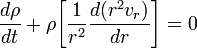$\frac{d\rho}{dt} + \rho \biggl[\frac{1}{r^2}\frac{d(r^2 v_r)}{dr} \biggr] = 0$

Euler Equation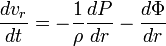$\frac{dv_r}{dt} = - \frac{1}{\rho}\frac{dP}{dr} - \frac{d\Phi}{dr}$

Poisson Equation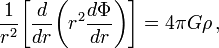$\frac{1}{r^2} \biggl[\frac{d }{dr} \biggl( r^2 \frac{d \Phi}{dr} \biggr) \biggr] = 4\pi G \rho \, ,$

but, in place of the adiabatic form of the 1st Law of Thermodynamics, we enforce isothermality both in space and time by adopting the,

Isothermal Equation of State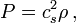$~P = c_s^2 \rho \, ,$

where,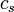$~c_s$, is the isothermal sound speed. This is equivalent to adopting an ideal-gas equation of state and setting,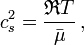$~c_s^2 = \frac{\mathfrak{R}T}{\bar\mu} \, ,$

then implementing the isothermality condition by holding the gas temperature constant. Recognizing that the differential element of mass that is contained within a spherical shell of width,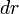$~dr$, at radial location,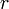$~r$, is,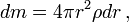$~dm = 4\pi r^2 \rho dr \, ,$

we see that the mass enclosed within radius,$~r$, is,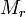$~M_r$$~=$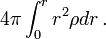$~4\pi \int_0^r r^2 \rho dr \, .$

Hence, we find from the Poisson equation that,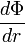$~\frac{d\Phi}{dr}$$~=$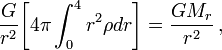$~\frac{G}{r^2} \biggl[ 4\pi \int_0^4 r^2 \rho dr \biggr] = \frac{GM_r}{r^2} \, ,$

which, when combined with the Euler equation gives the,

Combined Euler + Poisson Equation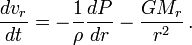$\frac{dv_r}{dt} = - \frac{1}{\rho}\frac{dP}{dr} - \frac{GM_r}{r^2} \, .$

In summary, then, the collapse of an isothermal gas cloud can be modeled by appropriately specifying an initial state and value of the sound speed, then integrating forward in time the following coupled set of equations:

Lagrangian Frame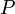$~P$$~=$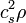$~c_s^2 \rho$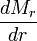$~\frac{dM_r}{dr}$$~=$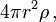$~4\pi r^2 \rho \, ,$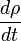$~\frac{d\rho}{dt}$$~=$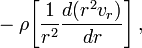$~- \rho \biggl[\frac{1}{r^2}\frac{d(r^2 v_r)}{dr} \biggr] \, ,$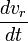$~\frac{dv_r}{dt}$$~=$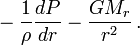$~- \frac{1}{\rho}\frac{dP}{dr} - \frac{GM_r}{r^2} \, .$

The lefthand side of the two equations containing time derivatives will take a different form if, alternatively, the choice is made to view the evolution from an Eulerian frame of reference. In this case the set of governing equations becomes,

Eulerian Frame$~P$$~=$$~c_s^2 \rho$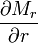$~\frac{\partial M_r}{\partial r}$$~=$$~4\pi r^2 \rho \, ,$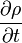$~\frac{\partial \rho}{\partial t}$$~=$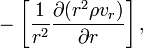$~- \biggl[\frac{1}{r^2}\frac{\partial (r^2 \rho v_r)}{\partial r} \biggr] \, ,$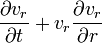$~\frac{\partial v_r}{\partial t} + v_r \frac{\partial v_r}{\partial r}$$~=$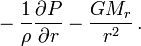$~- \frac{1}{\rho}\frac{\partial P}{\partial r} - \frac{GM_r}{r^2} \, .$

In either case, we can reduce the number of dependent variables by one — and, in conjunction, reduce the set of equations by one — by explicitly replacing$~P$ by the term on the righthand side of the isothermal equation of state, in which case the first term on the righthand side of the Euler + Poisson equation becomes,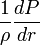$~\frac{1}{\rho} \frac{dP}{dr}$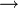$~~\rightarrow~~$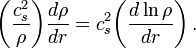$~\biggl( \frac{c_s^2}{\rho} \biggr) \frac{d\rho}{dr} = c_s^2 \biggl( \frac{d\ln\rho}{dr} \biggr) \, .$

## Governing Relations Adopted in Various Research Publications

Each of the papers referenced below presents results from an investigation into the development of nonlinear structure throughout the volume of a dynamically collapsing isothermal sphere. Here we demonstrate that, in each of these published studies, the coupled set of governing equations is the same as (either the Lagrangian or the Eulerian) set of equations that we have identified, above. The published results differ from study to study, either because …

• the adopted initial model configurations and/or adopted boundary conditions differ; or
• instead of seeking the solution of a particular initial value problem, the authors choose to examine in broad terms how the structure of the flow should develop, given the nature of the governing equations.

### Direct Numerical Simulation of the Initial Value Problem

#### Bodenheimer & Sweigart (1968)

As is detailed at the beginning of §II of their paper, Bodenheimer & Sweigart (1968, ApJ, 152, 515) adopt a Lagrangian Frame of reference. The coordinate,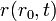$~r(r_0, t)$, is used to track over time the location of each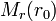$~M_r(r_0)$ mass shell whose initial radial location is,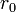$~r_0$. Hence,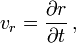$~v_r = \frac{\partial r}{\partial t} \, ,$

and the combined Euler + Poisson equation may be rewritten as (see their equation 1),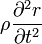$~\rho \frac{\partial^2 r}{\partial t^2}$$~=$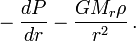$~- \frac{dP}{dr} - \frac{GM_r \rho}{r^2} \, .$

Bodenheimer & Sweigart (1968) recognize that, when viewed from this Lagrangian perspective, integration of the continuity equation would be redundant as conservation of mass is guaranteed by using the differential expression (see their equation 2),$~\frac{\partial M_r}{\partial r}$$~=$$~4\pi r^2\rho \, ,$

to track how the mass density varies as the coordinate location of each mass shell,$~r$, varies.

### Similarity Solution

#### Larson (1969)

In Appendix C of a seminal paper, Richard B. Larson (1969, MNRAS, 145, 271) presents an asymptotic similarity solution for the isothermal collapse of a sphere. He begins by stating that, in Eulerian form, the equations governing the isothermal collapse may be written (see his equation set C1),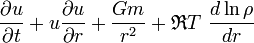$~\frac{\partial u}{\partial t} + u \frac{\partial u}{\partial r} + \frac{Gm}{r^2} + \mathfrak{R} T ~\frac{d\ln\rho}{d r}$$~=$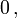$~0 \, ,$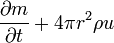$~\frac{\partial m}{\partial t} + 4\pi r^2\rho u$$~=$$~0 \, ,$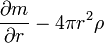$~\frac{\partial m}{\partial r} - 4\pi r^2 \rho$$~=$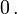$~ 0 \, .$

Apart from the different adopted variable names, it is clear that the first and third of these equations have exact counterparts in the set of "Eulerian Frame" governing equations that we have identified, above. The second equation replaces the continuity equation, providing a different but equally valid statement of mass conservation. It is most straightforwardly derived by recognizing that the quantity,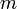$~m$, can be used as a Lagrangian tracer whose (Lagrangian) time-derivative is zero throughout an evolution, then switching to an Eulerian frame of reference. That is,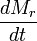$~\frac{dM_r}{dt}$$~=$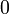$~0$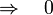$~\Rightarrow ~~~ 0$$~=$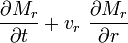$~\frac{\partial M_r}{\partial t} + v_r ~\frac{\partial M_r}{\partial r}$$~=$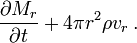$~\frac{\partial M_r}{\partial t} +4\pi r^2 \rho v_r \, .$

# See Especially

 © 2014 - 2021 by Joel E. Tohline |   H_Book Home   |   YouTube   | Appendices: | Equations | Variables | References | Ramblings | Images | myphys.lsu | ADS | Recommended citation:   Tohline, Joel E. (2021), The Structure, Stability, & Dynamics of Self-Gravitating Fluids, a (MediaWiki-based) Vistrails.org publication, https://www.vistrails.org/index.php/User:Tohline/citation